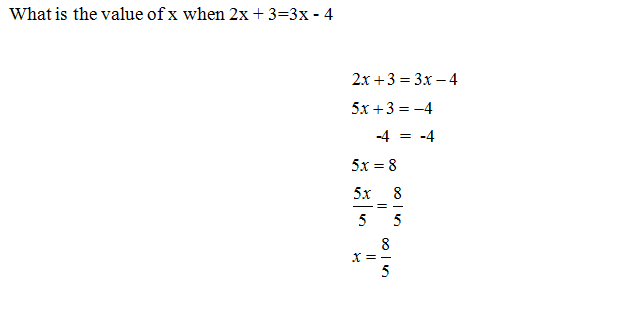Categories

# Solving EquationsThe student clearly needs more practice with solving equations. Fine. But why did the student make this mistake, in particular? After all, there are dozens of ways to mess up this problem. Why was this way tempting?

Today’s submissions comes Louise Wilson, who blogs over at Crazed Mummy.

## 5 replies on “Solving Equations”

John Achesonsays:

Students are programmed to add like terms and they often don’t understand the concept of transferrring or moving terms from one side of the equation to the other involves inverse operations. They tend to ignore the equal sign. I see this quite often in my classes as well. I like to show them what we are trying to do in isolating the variable and getting all of the variables gathered on the same side in order to do so. I revisit the inverse concept in order to achieve this.

mr bombasticsays:

Is there a typo in this? Where did the “8” come from?

A very common mistake once they get to 5x + 3 = -4 would be to add 4 to both sides, and then write 5x = 7. There thinking is that there is literally “nothing” on the right side instead of a “0”. But, they know they need an equal sign, so they just put it between the 5x & the 7. I know this, because students have told me that is what they were thinking on several occasions.

Matt Moransays:

I agree with mr bombastic about there being a very common mistake that students make in solving equations with like terms,but I think something else is going on here.

I wonder if this student may have thought that it would help to “undo” the -4 on the right side of the equation. I would view the mistakes with this problem as:
1) Incorrectly combining the 2x and 3x like terms to get 5x
2) Eliminating the -4 on the right side by combining with another -4
3) Treating the sum of -4 and -4 as +8 rather than -8
4) Ignoring the + 3 term on the left side once the -4 was “eliminated”

The error with the 2x and 3x terms shows the student does not understand how to manipulate like terms. Many students also fail to realize that you want to isolate the variable and do not know how or why to move terms without the variable to another side. When a student misunderstands this much, it often leads to some random guessing of strategies. That is why I suspect the student thought that he or she could “undo” the -4 by focusing on that term. From there, it seems likely to me that a student missing those concepts could also err on combining the -4 and -4 to make +8.

I don’t know how someone could arrive at this solution without making multiple mistakes, and given the error in combining 2x and 3x AND the strange +8 term, there must be 3 or more mistakes to arrive at this solution.

Kitsays:

One thing I use to help my students keep their solutions organized is that I have them draw a vertical line down their page through the equal sign. Then, when we see multiple variable terms in the equation, we discuss “are they on the same side of the line?” if so, then we know we just combine like terms. If they’re on opposite sides of the line, well, we know the only way to cross the line is using inverse operations, so we’ll use inverse operations to combine the like terms.

Juliesays:

This student knows that 2x and 3x needed to be place together, but you cannot just add them together, which is one of the mistakes this student made. They are in two separate equations that are equal to each other. If there wants an equal sign then 2x and 3x can be added together. This student knew that x’s needs to be added together, but the student does not understand how to terms work in a equation. I would help this student by making a new equation that is simpler for the student to understand before giving this student the problem above. For example, I would start with 2x + 1 – x = ?. Because this problem will help the student understand that you can combine the same terms together but he or she have to keep in mind that the minus sign affects the value of x as well. It is important for the student to understand the basic steps and rules of solving equation before moving on.